# Abelian group

## Definition

### Symbol-free definition

An abelian group is a group in which any two elements commute.

### Full definition

An abelian group is a set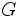equipped with a (infix) binary operation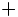(called the addition or group operation), an identity elementand a (prefix) unary operation, called the inverse map or negation map, satisfying the following:

• For any,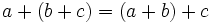. This property is termed associativity.
• For any,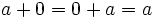.thus plays the role of an additive identity element or neutral element.
• For any,. Thus,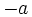is an inverse element towith respect to.
• For any,. This property is termed commutativity.

## Notation

Whenis an abelian group, we typically use additive notation and terminology. Thus, the group multiplication is termed addition and the product of two elements is termed the sum.

1. The infix operatoris used for the group multiplication, so the sum of two elementsandis denoted by. The group multiplication is termed addition and the product of two elements is termed the sum.
2. The identity element is typically denoted asand termed zero
3. The inverse of an element is termed its negative or additive inverse. The inverse ofis denoted.
4.donetimes is denoted, (where) whiledonetimes is denoted.

This convention is typically followed in a situation where we are dealing with the abelian groupin isolation, rather than as a subgroup of a possibly non-abelian group. If we are working with subgroups in a non-abelian group, we typically use multiplicative notation even if the subgroup happens to be abelian.

## Examples

### Some infinite examples

The additive group of integers, the additive group of rational numbers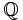, the additive group of real numbers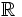, the multiplicative group of nonzero rationals, and the multiplicative group of nonzero real numbersare some examples of Abelian groups.

(More generally, for any field, the additive group, and the multiplicative group of nonzero elements, are abelian groups).

### Finite examples

Cyclic groups are good examples of abelian groups, where the cyclic group of orderis the group of integers modulo.

The additive group of the ring of integers, and more generally of any ring, is an abelian group.

### Arithmetic functions under Dirichlet product

Further information: Multiplicative functions form abelian group under Dirichlet product

The arithmetic functions, i.e., functions from the set of natural numbers to a commutative unital ring, admit a commutative and associative binary operation called the Dirichlet product. Those arithmetic functions whose value atis invertible are invertible with respect to this Dirichlet product.

The multiplicative functions form a subgroup of this group.

### Arithmetic functions under pointwise addition

The arithmetic functions from the natural numbers to a commutative unital ring form an abelian group under pointwise addition.